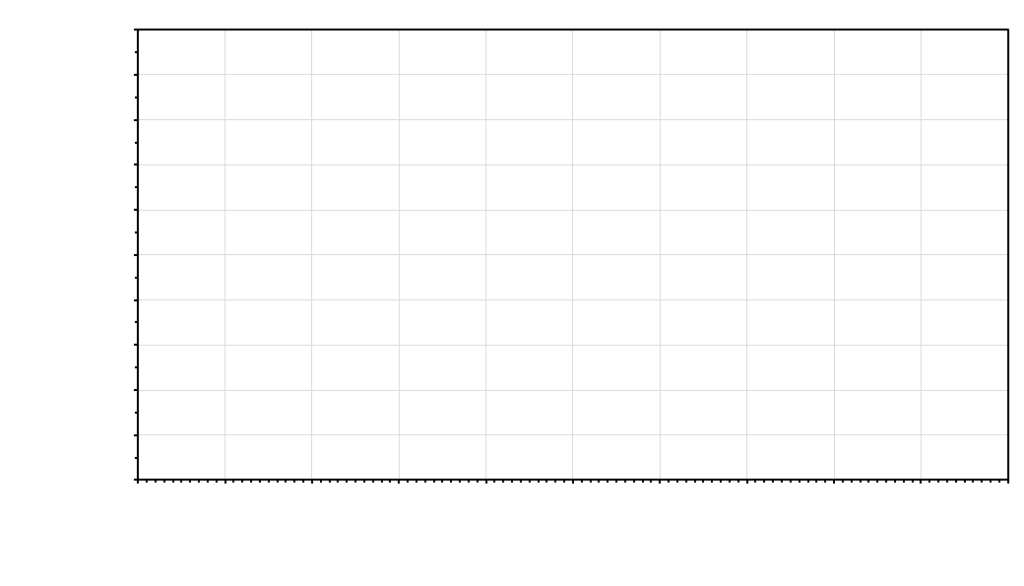## Running the experiment and recording data

The final experimental protocol collectively agreed upon will be written on the board. Start collecting data. All the data from all the students who volunteer to be measured will be pooled. You will plot the data for the entire class.

### Lab 3 Exercises 3.4

1. Write the data for your volunteers in the table, then write in data from the rest of the class. Use the computer provided to calculate body surface area from height and weight. Use the computer provided to calculate lung capacity from balloon circumference.
Height (cm) Weight (kg) Body surface area ($m^{2}$) Lung capacity

1. You have three hypotheses about which easily measured attribute best correlates with total lung capacity. In the three graphs below, plot the data set for each hypothesis. Under each graph, indicate whether the relationship provides evidence for accepting or rejecting the hypothesis.Hypothesis 1. Result. Accept or reject the hypothesis? Why?Hypothesis 2. Result. Accept or reject the hypothesis? Why?Hypothesis 3. The total surface area of individuals’ bodies will correlate well with their total lung capacity and total body surface area will correlate better with total lung capacity than any other easily measured body characteristic. Result. Accept or reject the hypothesis? Why?